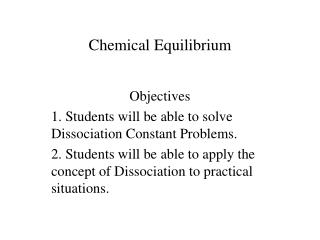# Chemical Equilibrium - PowerPoint PPT PresentationDownload PresentationChemical Equilibrium

Download Presentation## Chemical Equilibrium

- - - - - - - - - - - - - - - - - - - - - - - - - - - E N D - - - - - - - - - - - - - - - - - - - - - - - - - - -
##### Presentation Transcript

1. Chemical Equilibrium Objectives 1. Students will be able to solve Dissociation Constant Problems. 2. Students will be able to apply the concept of Dissociation to practical situations.

2. A + B  CThe above reaction does not always occur. In reality, there is a back and forth process.A + B  C, butC  A + B at the same time!

3. The rate for A +B  C is called the forward rate.The rate for C  A + B is called the reverse rate.

4. Quantitatively, the ratio of the forward rate to the reverse rate equals Q, the reaction Quotient. For any reactionaA + bBcC + dDQ=[C]c[D]d/[A]a[B]b[A],[B],[C], and [D] are the molarconcentrations of A,B,C, and D

5. When the forward and reverse reaction rates equal each other, a reaction is in equilibrium.K=[C]c[D]d/[A]a[B]bK is the equilibrium constant.

6. Le Chatelier’s Principle states that the concentrations of products and reactants will change such that the Reaction Quotient will equal K.If concentrations of products must increase, then reaction “shifts” right. If concentration of reactions must increase, then reaction “shifts” left.

7. For the Reaction A + B  2C + 2D K=1×102[A]=30M, [B]=20M, [C]=40M, [D]=50MWhat is Q?Q=[40M]2[50M]2/[30M][20M]Q=6.67×103, Concentrations of reactants must increase. Reaction “shifts” left.

8. How do we find the equilibrium concentrations?

9. A) Define variables (See what changes)Let x=amount of [A] formedLet x=amount of [B] formedLet 2x=amount of [C] lostLet 2x=amount of [D] lostLet +x=new amount of [A]Let +x=new amount of [B]Let -2x=new amount of [C]Let -2x=new amount of [D]

10. B) Solve for x1×102=[40-2x]2[50-2x]2/[30+x][20+x]x=[12.5M]new amount of A=[42.5M]new amount of B=[32.5M]new amount of C=[15M]new amount of D=[25M]

11. Summary of Problem Solving Steps1) Determine value of Q2) Determine variables3) Determine values of variablesSuppose that Q already equals K?What should we do?

12. Take-home concepts:Define forward and reverse rate,the differences between Q and K,and Le Chatelier’s Principle.